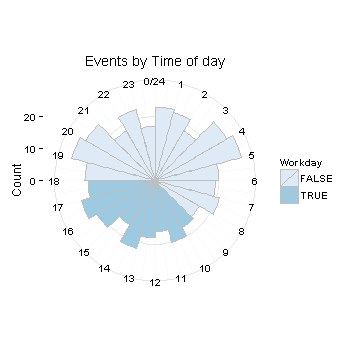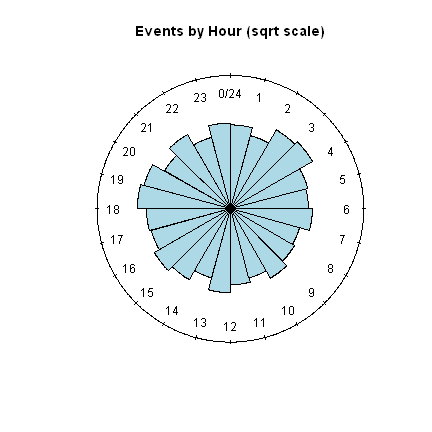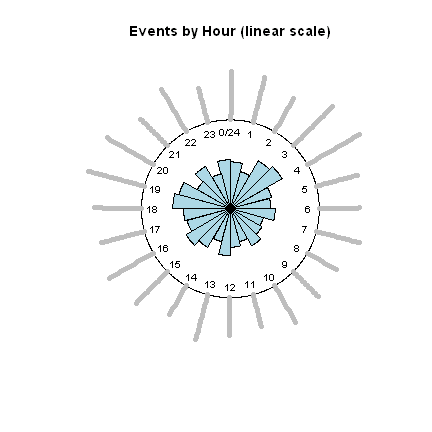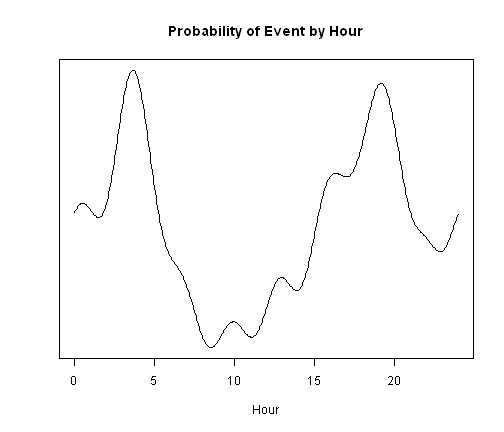# Circular time plots in R

Circular plots can help us to see patterns of activity that depend on the time of day. This is a short demonstration of visualizing events by time-of-day using ggplot2 and circular packages. We'll also use lubridate to work with dates/times. This was inspired by a response on stackoverflow. It's also partly an excuse to try out R Markdown.

First, let's load our packages and generate some random event data

``````library(lubridate)
library(ggplot2)   # use at least 0.9.3 for theme_minimal()

## generate random data in POSIX date-time format
set.seed(44)
N=500
events <- as.POSIXct("2011-01-01", tz="GMT") +
days(floor(365*runif(N))) +
hours(floor(24*rnorm(N))) +  # using rnorm here
minutes(floor(60*runif(N))) +
seconds(floor(60*runif(N)))
``````

Next, we organize the events a little. We're going to bin the events by hour and, to add some color, we'll categorize events based on whether they were during the 'workday' (defined here as 9 thru 5pm).

``````# extract hour with lubridate function
hour_of_event <- hour(events)
# make a dataframe
eventdata <- data.frame(datetime = events, eventhour = hour_of_event)
# determine if event is in business hours
eventdata\$Workday <- eventdata\$eventhour %in% seq(9, 17)
``````

### Plotting with ggplot2 package

Our first method of plotting is from ggplot2. I am a fan of `theme_minimal()`, which is new to ggplot2 0.9.3. If you are using an older version, you can substitute `theme_bw()` below.

``````ggplot(eventdata, aes(x = eventhour, fill = Workday)) + geom_histogram(breaks = seq(0,
24), width = 2, colour = "grey") + coord_polar(start = 0) + theme_minimal() +
scale_fill_brewer() + ylab("Count") + ggtitle("Events by Time of day") +
scale_x_continuous("", limits = c(0, 24), breaks = seq(0, 24), labels = seq(0,
24))
``````Easy and lovely!

### Plotting with circular package

Other alternative plotting methods can be found in the package circular. These seem a little more fussy compared to ggplot2 in that plots required hand-tuning to make them look reasonable. In particular, I needed to play with the `prop` argument, which is a numerical constant determining the radii of the sectors, and `shrink`, which controls the size of the plotted circle. And I never did figure out how to make it display bins in different colors.

``````library(circular)
``````
``````## Attaching package: 'circular'
``````
``````## The following object(s) are masked from 'package:stats':
##
## sd, var
``````
``````
# make our data into circular class from package circular
eventdata\$eventhour <- circular(hour_of_event%%24, # convert to 24 hrs
units="hours", template="clock24")
``````
``````# plot a rose diagram, setting prop(ortion) argument after trial-n-error
rose.diag(eventdata\$eventhour, bin = 24, col = "lightblue", main = "Events by Hour (sqrt scale)",
prop = 3)
``````The default for `rose.diag()` is to display the squareroot of the values being plotted, which is often helpful for visualizing counts. We can also make the scale linear if we want the peaks to really stand out by setting `radii.scale = "linear"`. Also, `rose.diag()` lets us add points to the surface of our plot, which is a little redundant here, but which could be useful if we had another variable to display:

``````# redundantly add points to surface; we need to adjust parameters like
# shrink, cex, and prop
rp <- rose.diag(eventdata\$eventhour, bin = 24, col = "lightblue", main = "Events by Hour (linear scale)",
prop = 12, radii.scale = "linear", shrink = 1.5, cex = 0.8)
points(eventdata\$eventhour, plot.info = rp, col = "grey", stack = TRUE)
``````Finally, we can estimate the density and plot it more conventionally. The function `circular.density()` is needed since our data “wrap around”. A problem with density plots is that your average person can't read the y-axis, so let's just hide the numbers with `yaxt='n'` and try to clearly label the plot instead.

``````# estimate density, class is still circular not density
bw <- 10 * bw.nrd0(eventdata\$eventhour)  # may not be best bw: experiment
dens <- density.circular(eventdata\$eventhour, bw = bw)  # bw must be given
``````
``````# returns NULL for some reason
plot(dens, plot.type = "line", join = TRUE, main = "Probability of Event by Hour",
xlab = "Hour", ylab = "", yaxt = "n")
````````````## NULL
``````